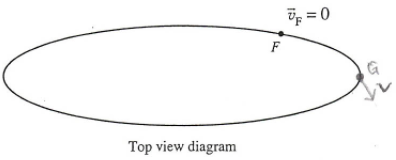# Problem: An object starts from rest at point F and speeds up continuously as it moves around an oval.a. Choose a point about 1/8th of the way around the oval from point F, and label it point G. Draw a vector to represent the velocity of the object at point Gb. Determine the change in velocity vector between points F and Gc. How would you characterize the direction of Δv as point G moves closer and closer to point F?d. Each of the following statements in incorrect. Discuss the flaws in the reasoning.i. “The acceleration at point F is zero. As point G becomes closer and closer to point F, the change in velocity vector becomes smaller and smaller. Eventually, it becomes zero.”ii. “The acceleration at point F is perpendicular to the curve”

###### FREE Expert Solution

a.

The velocity vector of the object at point G is the tangent along the path.

88% (34 ratings)###### Problem Details

An object starts from rest at point F and speeds up continuously as it moves around an oval.

a. Choose a point about 1/8th of the way around the oval from point F, and label it point G. Draw a vector to represent the velocity of the object at point G

b. Determine the change in velocity vector between points F and G

c. How would you characterize the direction of Δv as point G moves closer and closer to point F?

d. Each of the following statements in incorrect. Discuss the flaws in the reasoning.

i. “The acceleration at point F is zero. As point G becomes closer and closer to point F, the change in velocity vector becomes smaller and smaller. Eventually, it becomes zero.”

ii. “The acceleration at point F is perpendicular to the curve”